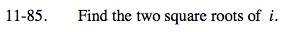### Home > PC > Chapter 11 > Lesson 11.2.2 > Problem11-85

11-85.If you were to graph i in the complex plane, it would be located at (0, 1).What are the polar coordinates for the point (0, 1)?

$\textit{r} = 1, \theta=\frac{\pi}{2}, \textit{n}=2$

For k = 0, 1.

$\sqrt{1}\left( \cos\left( \frac{\frac{\pi}{2}+2\textit{k}\pi}{2 \right) +\textit{i} \sin\left( \frac{\frac{\pi}{2}+2\textit{k}\pi}{2 \right) \right)$

k = 0

$\cos\frac{\pi}{4}+\textit{i}\sin\frac{\pi}{4}-\frac{\sqrt{2}}{2}+\frac{\sqrt{2}}{2}\textit{i}$

k = 1

$\sqrt{1}\left( \cos\left( \frac{\frac{\pi}{2}+2\pi}{2 \right) +\textit{i} \sin\left( \frac{\frac{\pi}{2}+2\pi}{2 \right) \right) \left( \cos\frac{5\pi}{4} +\textit{i} \sin\frac{5\pi}{4} \right) ? ?$